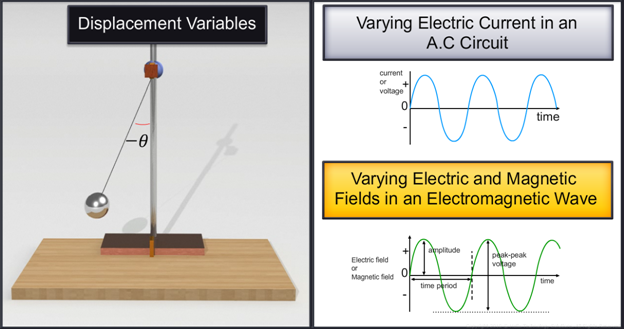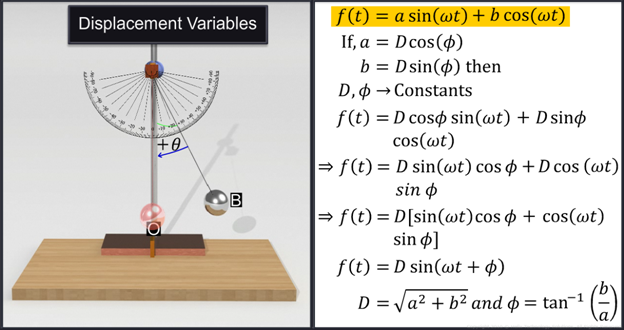Notes On Period, Frequency and Displacement - CBSE Class 11 Physics
All oscillatory motions like that exhibited by a pendulum are periodic motions. The smallest regular fixed interval of time after which the motion of a body in oscillatory motion repeats itself is called the periodic time or time period denoted by T. Time period T is measured in seconds.   Frequency is defined as the number of oscillations made by the oscillating particle or a body in a unit time. It is denoted by the letter ‘n’.   Frequency is equal to the reciprocal of time period T.   Frequency (n) = 1/ time period (T)   T = 1/n or n = 1/T   Frequency is measured in cycles per second (cps) or hertz and is denoted by the letters ‘Hz’ named after the scientist Heinrich Rudolf Hertz.   When the pendulum moves away from the mean position O towards the extreme position its displacement from the mean position increases and when the pendulum returns towards O, the displacement from the mean position decreases.   In oscillatory motion, all displacements are measured from the mean position. The displacement can be positive or negative after fixing the reference position.For example, if the same pendulum were to oscillate towards the left of the mean position O, its displacement can be taken as negative considering the right side of the reference position as positive. The displacement variable in general can be understood as the variations in physical quantity with time in a broader sense when the variable involved oscillates.     The varying electric current in an AC circuit and varying electric and magnetic fields in an electromagnetic wave can be considered as displacement variables.   a periodic function that is a combination of a sine and a cosine function. The importance of these two harmonic functions is due to the mathematical result proved by French mathematician Jean Baptiste Joseph Fourier. According to the result proved, any periodic function can be expressed as a superposition of sine and cosine functions with suitable coefficients as shown below.#### Summary

All oscillatory motions like that exhibited by a pendulum are periodic motions. The smallest regular fixed interval of time after which the motion of a body in oscillatory motion repeats itself is called the periodic time or time period denoted by T. Time period T is measured in seconds.   Frequency is defined as the number of oscillations made by the oscillating particle or a body in a unit time. It is denoted by the letter ‘n’.   Frequency is equal to the reciprocal of time period T.   Frequency (n) = 1/ time period (T)   T = 1/n or n = 1/T   Frequency is measured in cycles per second (cps) or hertz and is denoted by the letters ‘Hz’ named after the scientist Heinrich Rudolf Hertz.   When the pendulum moves away from the mean position O towards the extreme position its displacement from the mean position increases and when the pendulum returns towards O, the displacement from the mean position decreases.   In oscillatory motion, all displacements are measured from the mean position. The displacement can be positive or negative after fixing the reference position.For example, if the same pendulum were to oscillate towards the left of the mean position O, its displacement can be taken as negative considering the right side of the reference position as positive. The displacement variable in general can be understood as the variations in physical quantity with time in a broader sense when the variable involved oscillates.     The varying electric current in an AC circuit and varying electric and magnetic fields in an electromagnetic wave can be considered as displacement variables.   a periodic function that is a combination of a sine and a cosine function. The importance of these two harmonic functions is due to the mathematical result proved by French mathematician Jean Baptiste Joseph Fourier. According to the result proved, any periodic function can be expressed as a superposition of sine and cosine functions with suitable coefficients as shown below.Previous
Next